# 词云(Wordcloud)使用简单介绍2019年8月7日07:39:48

7 7021字阅读23分24秒

## 简介## 简单使用方式介绍

### 关于字体说明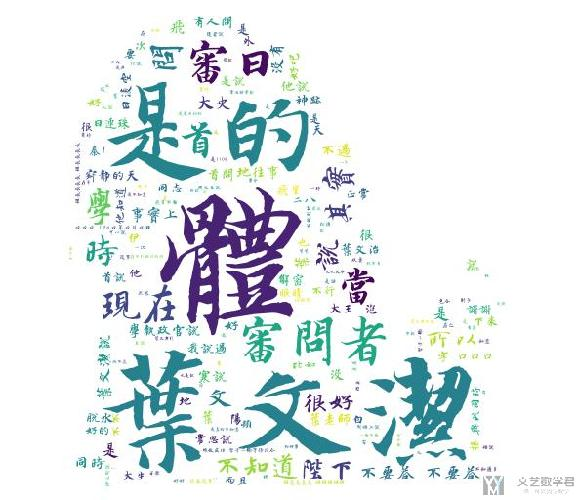### 使用前准备

1. from os import path
2. from wordcloud import WordCloud
3. from PIL import Image
4. import numpy as np
5. d = path.dirname(__file__)
6. # Read the whole text.
7. text = open(path.join(d, 'constitution.txt'), encoding='utf8').read()
8. # 导入字体文件
9. font_path = path.join(d, 'HYC6GFM.TTF')

### 生成普通的wordcloud

1. # 生成普通的wordcloud
2. wordcloud = WordCloud(font_path=font_path, margin=1, random_state=1, max_words=300, width=1000, height=700, background_color='white').generate(text)
3. wordcloud.to_file('wordcloud.jpg')### 使用蒙版来生成图片2. mask = np.array(Image.open(path.join(d, "62.jpg")))
3. wordcloud = WordCloud(font_path=font_path, mask=mask, margin=1, random_state=1, background_color='white').generate(text)## 关于中文文档的词云生成（例子）

### 中文句子分词

1. # 对句子进行分词
2. def seg_sentence(sentence):
3.     sentence_seged = jieba.cut(sentence.strip())  #strip()用来消除前后的空格
4.     outstr = ''
5.     for word in sentence_seged:
6.         if word != '\t':
7.             outstr += word
8.             outstr += " " #去掉制表符并用空格分隔各词
9.     return outstr.strip()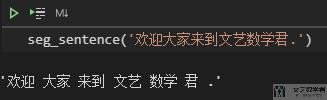### 停用词的处理

1. # 创建停用词list
2. def stopwordslist(filepath):
3.     stopwords = [line.strip() for line in open(filepath, 'r', encoding='utf-8').readlines()]
4.     return stopwords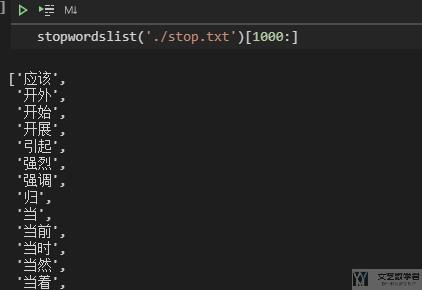### 中文句子分词与停用词

1. def seg_sentence(sentence):
2.    sentence_seged = jieba.cut(sentence.strip())
3.    stopwords = stopwordslist('./stop.txt')  # 这里加载停用词的路径
4.    outstr = ''
5.    for word in sentence_seged:
6.        if word not in stopwords: # 判断如果不是停用词
7.            if word != '\t':
8.                outstr += word
9.                outstr += " "
10.    return outstr

### 对中文文档绘制 wordcloud

1. import jieba
2. from wordcloud import WordCloud
3. import matplotlib.pyplot as plt
4. import numpy as np
5. from PIL import Image
6. inputs = open('./example.txt', 'r', encoding='utf-8') # 原始的中文文档
7. outputs = open('./output.txt', 'w', encoding='utf-8') # 分词过后的中文文档
8. for line in inputs:
9.     line_seg = seg_sentence(line)  # 对每个句子进行分词
10.     outputs.write(line_seg + '\n')  # 将处理过后的文件进行保存
11. outputs.close()
12. inputs.close()
13. mask = np.array(Image.open("./66.jpg")) # 模板图片
14. inputs = open('output.txt', 'r', encoding='utf-8')
17.                     max_words=300, min_font_size=10, max_font_size=None, repeat=False,
18.                     font_path="./FZKaTong-M19S.ttf").generate(mytext) #生成云图
19. wordcloud.to_file('wordcloud.jpg')
20. inputs.close()### 指定颜色风格

1. wordcloud=WordCloud(mask=mask, width=800, height=800, background_color="white", margin=1, max_words=10000, min_font_size=10, max_font_size=None, repeat=True,collocations=Falsemode='RGBA', colormap='Reds',font_path="./FZKaTong-M19S.ttf").generate(mytext)

## 词云与原图融合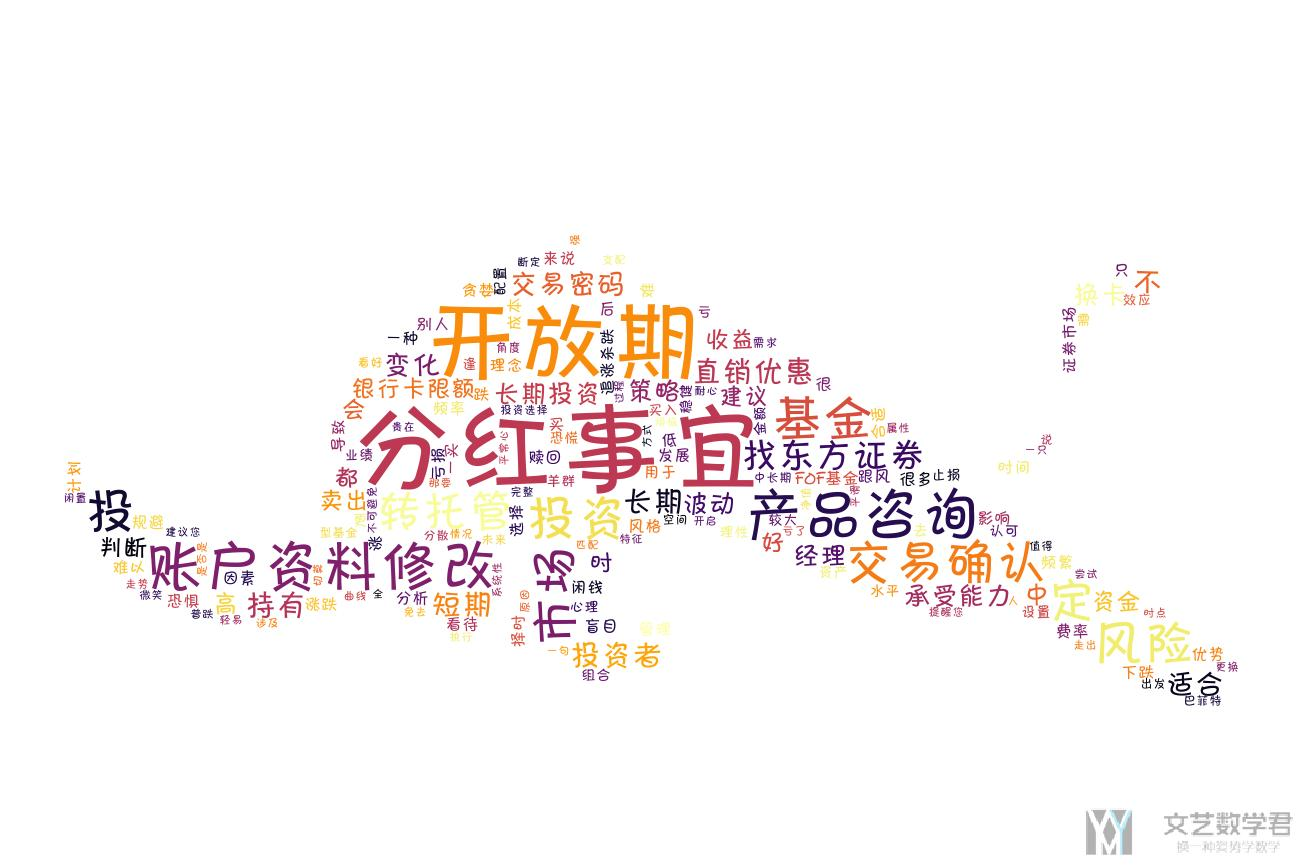1. img = Image.open('./niu_2.png')
2. background = Image.open('niu_1.jpg')
3. background = background.convert('RGBA') # 两个图片的格式需要相同
4. img = Image.blend(background, img, 0.7)
5. img.save('out_with_background.png')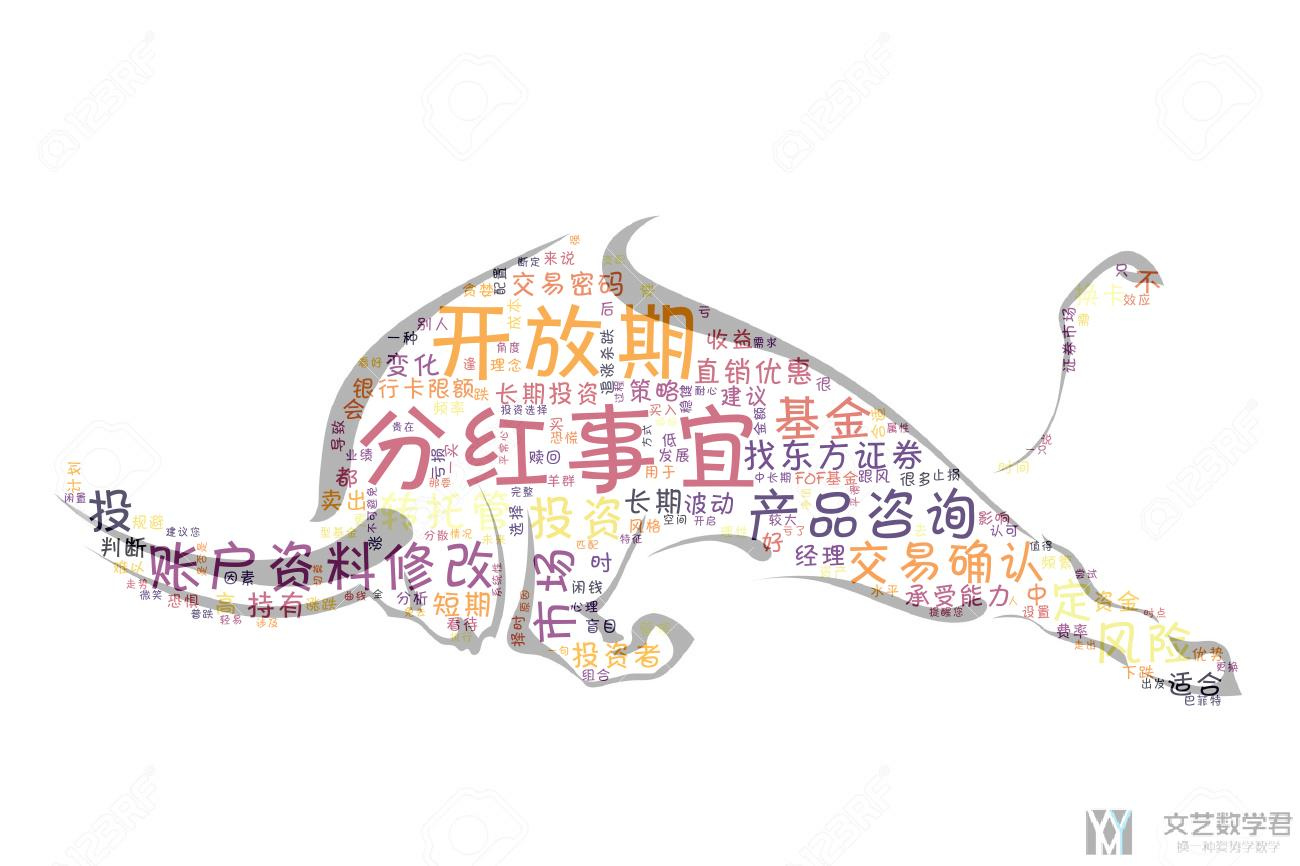## 词云与遮罩-进一步说明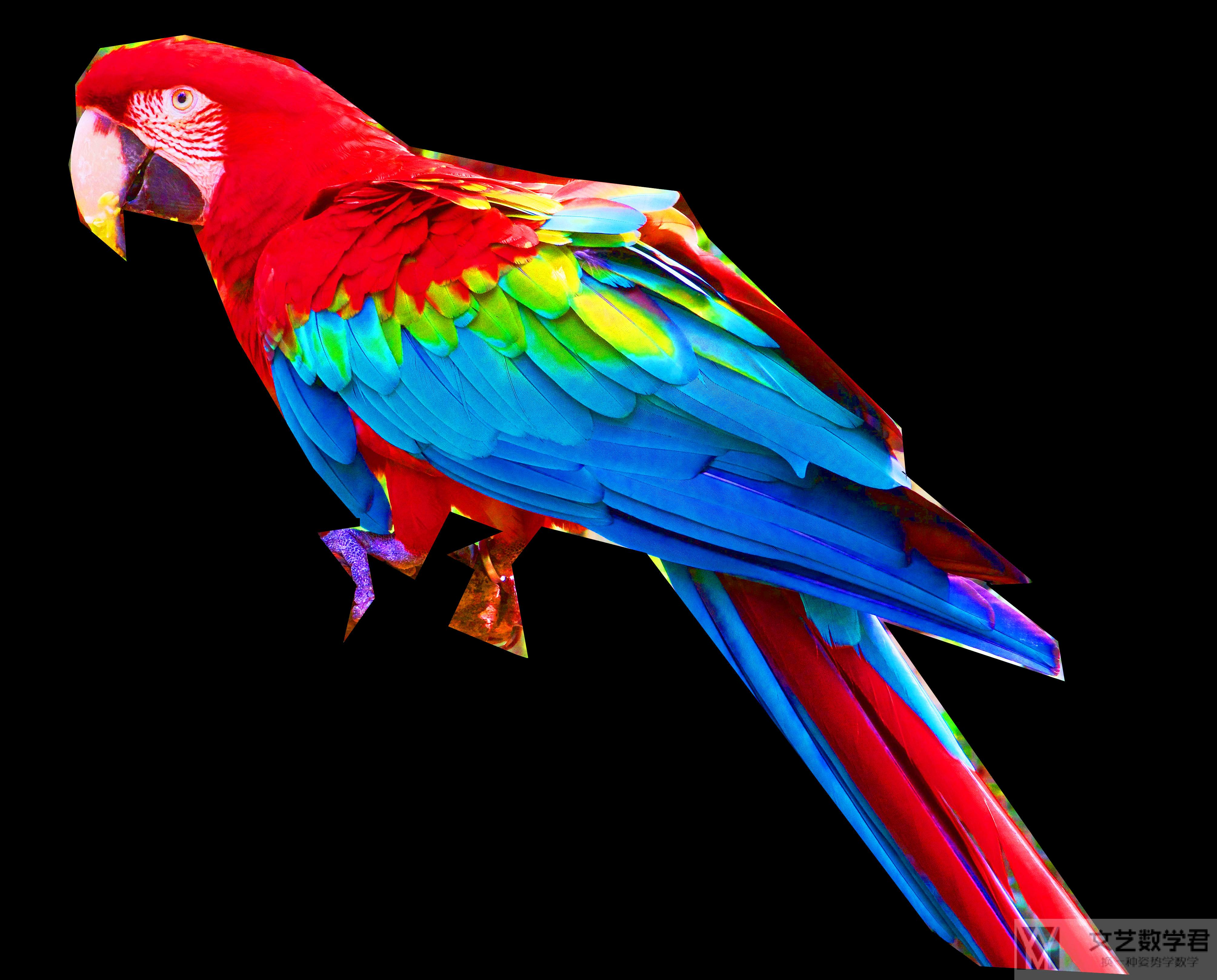### 图片的处理

1. from scipy.ndimage import gaussian_gradient_magnitude
2. # 图片的处理
3. img = Image.open("./parrot-by-jose-mari-gimenez2.jpg")
4. mask_color = np.array(img)
7. mask_image[mask_image.sum(axis=2) == 0] = 255 # 把黑色都转换为白色
8. # Edge detection
9. edges = np.mean([gaussian_gradient_magnitude(mask_color[:, :, i]/255., 2) for i in range(3)], axis=0)
10. mask_image[edges > .08] = 255
11. im = Image.fromarray(mask_image)
12. im.save("./your_file.jpeg")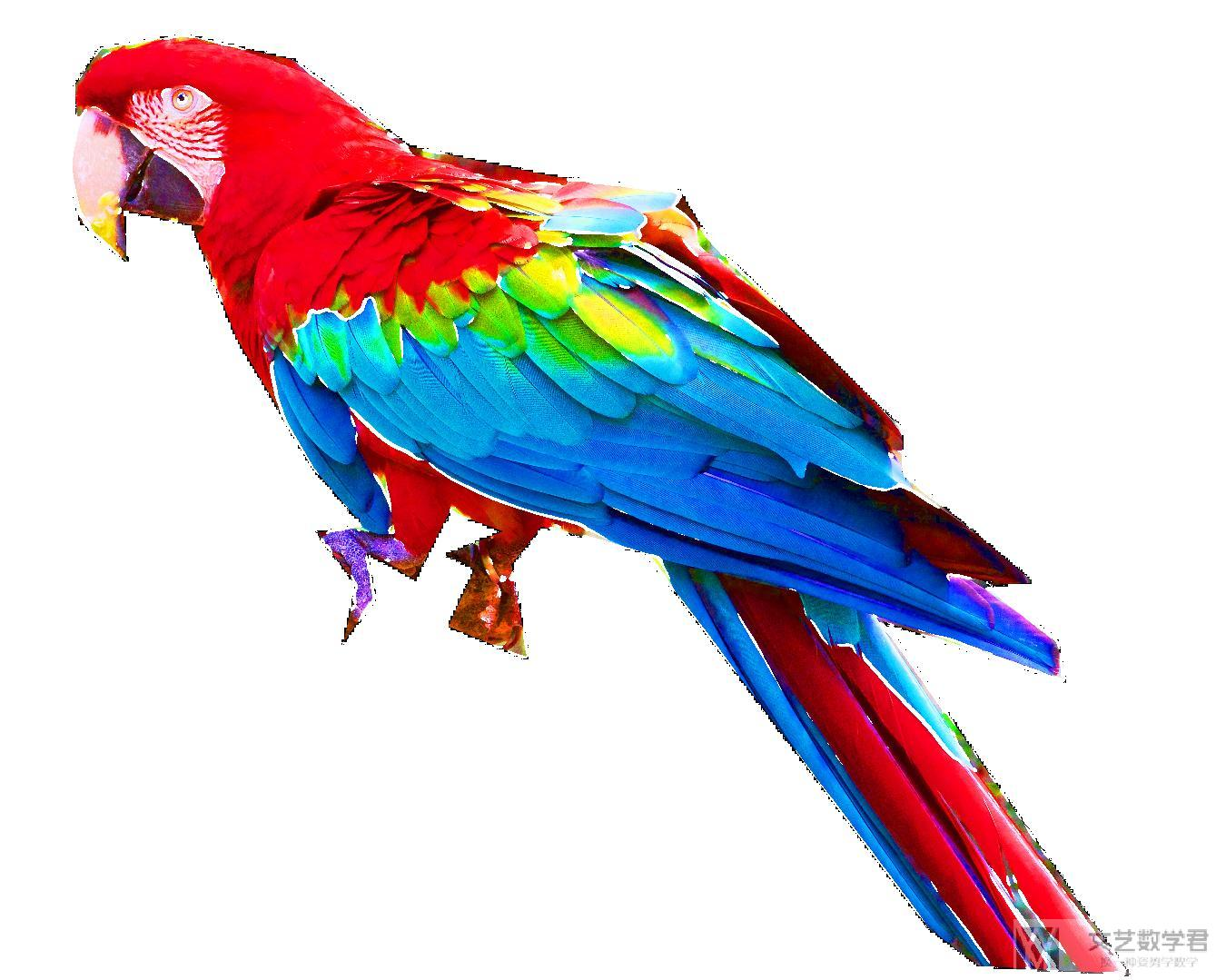### 简单的词云图像生成

1. # 绘制词云
2. inputs = open('./output.txt', 'r', encoding='utf-8')
5.                     max_words=10000, min_font_size=10, max_font_size=None, repeat=True,collocations=False,
6.                     mode='RGB',font_path="./FZKaTong-M19S.ttf").generate(mytext) #生成云图
7. wordcloud.to_file('wordcloud.jpg')
8. inputs.close()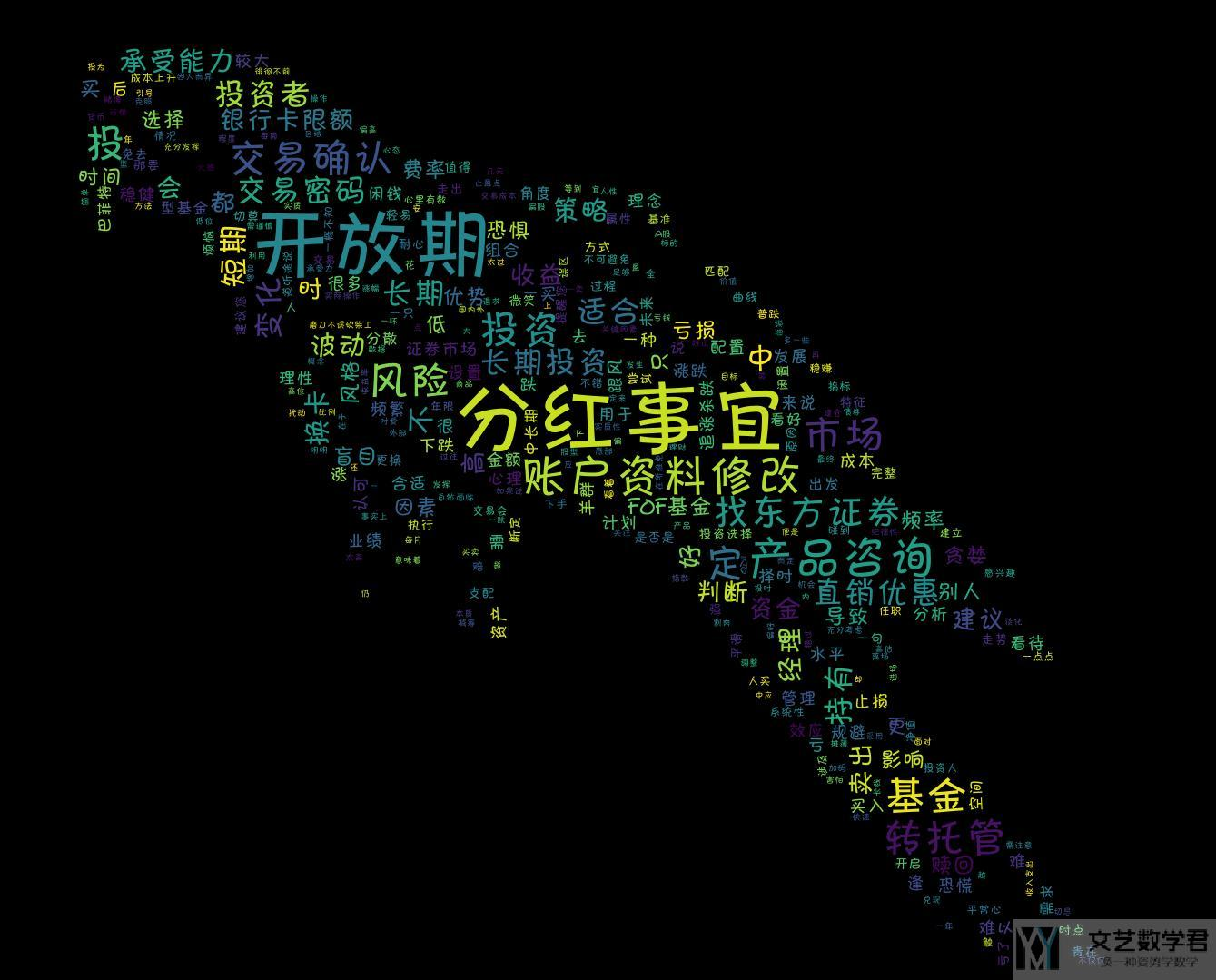### 产生特点颜色和形状的图云

1. # 绘制词云
2. inputs = open('./output.txt', 'r', encoding='utf-8')
5.                     max_words=10000, min_font_size=10, max_font_size=None, repeat=True,collocations=False,
6.                     mode='RGB',font_path="./FZKaTong-M19S.ttf").generate(mytext) #生成云图
7. # Create coloring from image
8. image_colors = ImageColorGenerator(mask_color)
9. wordcloud.recolor(color_func=image_colors)
10. # 保存至图片
11. wordcloud.to_file('wordcloud.jpg')
12. inputs.close()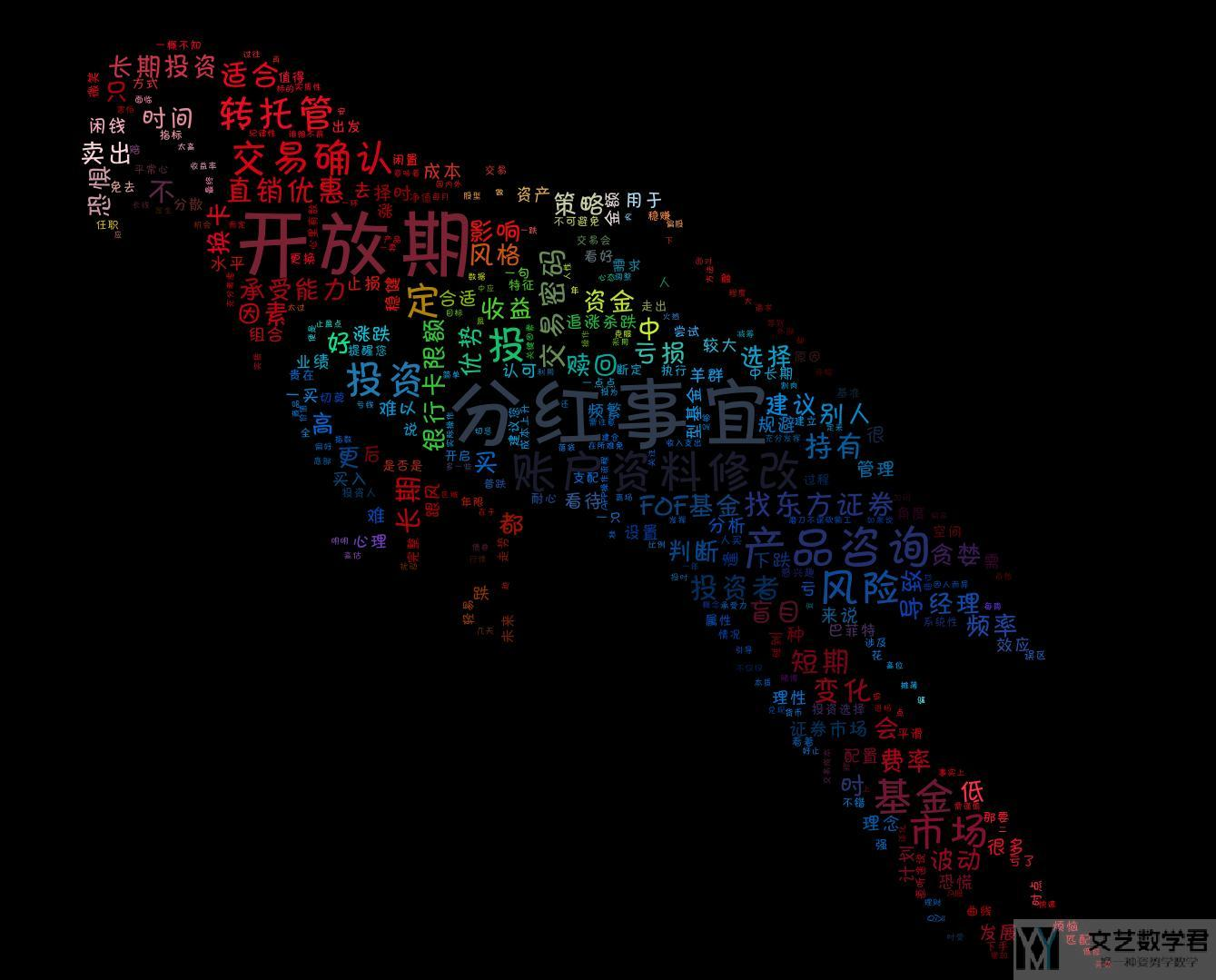1. import numpy as np
2. from PIL import Image
3. from wordcloud import WordCloud, ImageColorGenerator
4. from scipy.ndimage import gaussian_gradient_magnitude
5. # 图片的处理
6. img = Image.open("./parrot-by-jose-mari-gimenez2.jpg")
7. mask_color = np.array(img)
10. mask_image[mask_image.sum(axis=2) == 0] = 255 # 把黑色都转换为白色
11. # Edge detection
12. edges = np.mean([gaussian_gradient_magnitude(mask_color[:, :, i]/255., 2) for i in range(3)], axis=0)
13. mask_image[edges > .08] = 255
14. # 绘制词云
15. inputs = open('./output.txt', 'r', encoding='utf-8')
18.                     max_words=10000, min_font_size=10, max_font_size=None, repeat=True,collocations=False,
19.                     mode='RGB',font_path="./FZKaTong-M19S.ttf").generate(mytext) #生成云图
20. # Create coloring from image
21. image_colors = ImageColorGenerator(mask_color)
22. wordcloud.recolor(color_func=image_colors)
23. # 保存至图片
24. wordcloud.to_file('wordcloud.jpg')
25. inputs.close()

• 微信公众号
• 关注微信公众号
•• QQ群
• 我们的QQ群号
•• 本文由 发表于 2019年8月7日07:39:48
• 转载请务必保留本文链接：https://mathpretty.com/10951.html